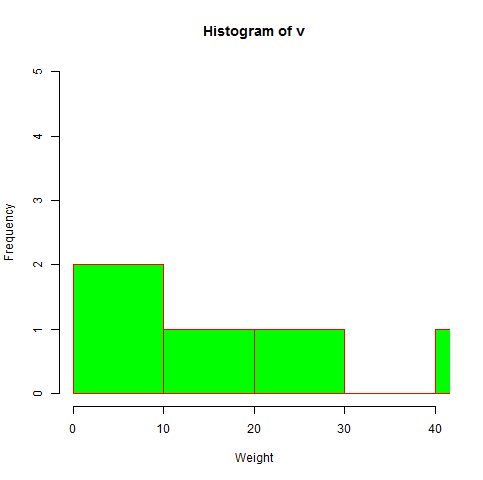R语言 直方图

R语言使用hist()函数创建直方图。 此函数使用向量作为输入，并使用一些更多的参数来绘制直方图。

语法

```hist(v,main,xlab,xlim,ylim,breaks,col,border)
```

• v是包含直方图中使用的数值的向量。
• main表示图表的标题。
• col用于设置条的颜色。
• border用于设置每个条的边框颜色。
• xlab用于给出x轴的描述。
• xlim用于指定x轴上的值的范围。
• ylim用于指定y轴上的值的范围。
• break用于提及每个条的宽度。

例

```# Create data for the graph.
v <-  c(9,13,21,8,36,22,12,41,31,33,19)

# Give the chart file a name.
png(file = "histogram.png")

# Create the histogram.
hist(v,xlab = "Weight",col = "yellow",border = "blue")

# Save the file.
dev.off()
```X和Y值的范围

```# Create data for the graph.
v <- c(9,13,21,8,36,22,12,41,31,33,19)

# Give the chart file a name.
png(file = "histogram_lim_breaks.png")

# Create the histogram.
hist(v,xlab = "Weight",col = "green",border = "red", xlim = c(0,40), ylim = c(0,5),
breaks = 5)

# Save the file.
dev.off()
```